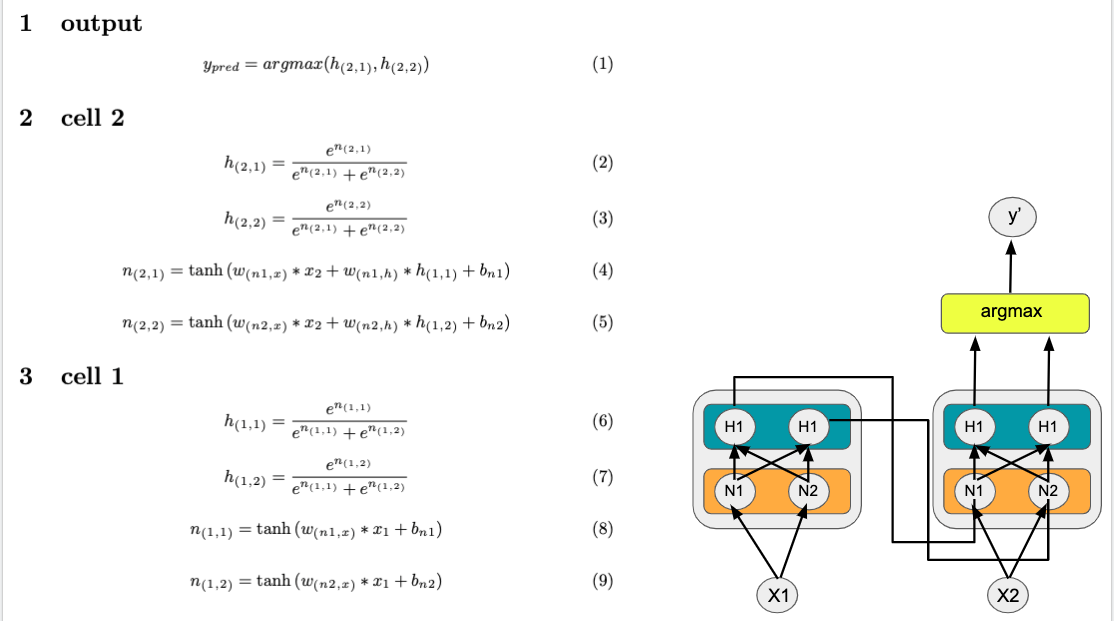# #StackBounty: #neural-networks #python #rnn #backpropagation #numpy Basic RNN sequence classifier diagram?

### Bounty: 50

I’d like to build an RNN in numpy from scratch to really get come comfortable with backpropagation through time (BPTT.) In the below diagram and LaTeX, I show two neurons, each with a non-linearity, N(i,j) and softmax/hidden state layer H(i,j).

The first neuron will receive x1, which will be sent to the non-linearities N1 and N2 (see equations 8 and 9 on left below); then, the N1 and N2 outputs will be sent to a softmax layer (see equations 6 and 7.)

In the following step, x2 will be sent to the second neuron; likewise, the h(1,1) and h(1,2) hidden state outputs will be sent as additional inputs to the second neuron. The nonlinearities will act upon these inputs (see eq 4 and 5) then be delivered to the final softmax layer, h(2,1) and h(2,2) (see eq 2 and 3.)

Lastly, argmax is applied to these hidden states and a predicted y value is returned (which is to say, the sequence label.)Because I want to implement the above from scratch, I will need to derive the gradients here. But before I move onto that step, I would like to know for certain that:
(A) The diagram respects a valid RNN, which can label sequences. and (B) that the equations on the left accurately depict what the diagram details.

To answer this equation, either confirm A and B (or if necessary, please provide guidance on what needs altering to achieve the stated effect.)

Edit: I took a stab at the gradients, however, I’m not sure of their accuracy.

Get this bounty!!!

This site uses Akismet to reduce spam. Learn how your comment data is processed.